# Drone altimeter by image analysis

For many drone airplane pilots, it is interesting to know how high the drone is flying. The following video explains the basics of the measuring method using image analysis.

The height measurement above ground is a distance measurement of the (vertical) distance from the ground. There are some tried and tested sensors for this purpose:

Often used
• Barometric altimeter
• GPS altimeter

Less often used (excerpt)
• Ultrasonic Distance Measurement
• laser triangulation
• Laser - Distance measurement by run-time

Measure altimeter is even without these sensors possible
But what do the pilots who do not have such sensors in the drone? Many pilots have a digital camera that can be lifted by the drone. For model airplanes, helicopters or drone may this camera not be too heavy. An action camera such as a GoPro camera can also be lifted by smaller models in the air. If you take a camera with the drone aircraft after the flight measurements can be carried out with the image information. The small camera is rotated so that the viewing direction is down. At the ground an object with known dimensions (eg a blanket) is placed and taken from the air. Subsequently the dimensions in pixels is determined on the computer. From these data the flight altitude or the distance of the camera can be calculated from the object.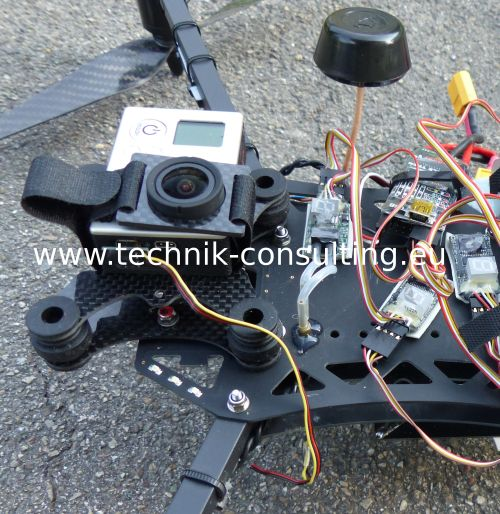Camera at the drone, view direction down in flight.

Even the drone can be a measurement object
For larger drone aircraft the camera can be positioned at the ground. The measurement object would then be for example the wingspan of the aircraft. For greater heights (depending on the drone from about 50 meters), this measurement method is too imprecise. It is better to carry the camera like a GoPro actioncam in the drone. The measurement object at the bottom can be easily enlarged to the expected flight altitudes as vice versa.

What is the height measurement by image analysis?
Put simply a known object is used as a reference and is calculated by the distance mathematics.

## Advantages against conventional sensors for height measurement

The image measurement offers numerous advantages over other sensors:
• Independence from satellite (GPS) and air pressure (barometric altitude).
• On the proper interpretation (calculations below) very precisely.
• High measuring rate depending on the camera is possible. Up to 50 measurements per second for the most cameras are not a problem.

To determine the height of the drone the camera has to be directed straight down. In addition an object of known dimensions is needed. This may be n picnic blanket, a towel or better a round tablecloth. If the model aircraft is over the well-known object it is possible to calculate the distance or height by simple geometrical calculations. The farther the distance to the (measurement) object so much smaller on the screen.

How small the blanket with the height or distance is can be calculated.

## Description of the height measurement on a drone

sketchD: Size of the object
h: height or distance of the camera to the object
ß: angle of vision of the object

The mathematical relationship is: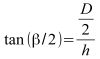With this arrangement the angle can also be calculated as follows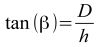Note: The triangle is not a right angle one.

The viewing angle can be calculated from a digital image as follows.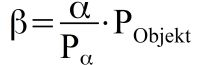ß: angle of vision of the object
A: angle of the camera
P_a: dimension of the screen diagonals of recording in pixels (on the angle of view is based on.)
P_Objekt: dimension of the object in pixels

The angle of view you can see from the data sheet at a fixed focus camera (eg GoPro cameras) often.

It is better if you determine the angle of vision. This is not difficult. The calculated angle of view is designated by "B". The following values can assume x 1020 pixels with a GoPro 3 at 1920 pixels: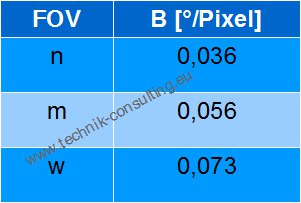The value of "B" indicates which visual angle corresponds to one pixel in degrees. The smaller this value, the larger the object will appear in the image.

## Camera as a rangefinder

Due to tolerances in the production but it is better to determine the angle of your camera itself and to calculate the value of "B".

Pick an object whose size and distance you know and make a picture of it. Suitable is for example a round traffic sign, a rectangular window frame or another high-contrast object. Measure from this and take a picture of this object from a known distance. A video recording is also possible when you create still images later. Ensure that the object is located roughly in the middle of recording. Some lenses distort the picture in the edge region, which is why this area is inappropriate.

For the calculation of the following equation applies. Exactly this value you calculate or use the table below.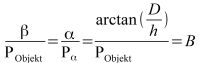example
A window frame with 85 cm at a distance of 30 meters on the image shows  34 Pixel ? 0,048 °/Pixel. This value will be denoted by B. B is different from camera to camera, and should always be determined at the start.

## Trust this type of altimeter measurement

Through the measurements of known objects you quickly gain confidence in this measurement method. The distance or the height you determined by the formula. Make sure when calculating with a calculator that the angle function is set to degrees ("DEG").

## Simple calculation of the distance or the height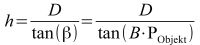B: The visual angle of one pixel. Depending on the receiving system. The calculation is explained above.
P_Objekt: dimensions of the known measurement object in pixels
D: The size of the known measurement object in meters.

The reference object may be a round tablecloth or similar. If necessary, a towel, a car etc.. Flat objects are better than high.

Thus, you can easily calculate the altitude of your aircraft (eg drone, quadcopter, helicopter or airplane). This also works in reverse, if you photograph your aircraft from the ground. Unfortunately, the limits are quickly reached with small drones, which is why at greater distances (approximately from 50 meters, depending on the drone) is the other way better. You can scale the object on the ground and thus additionally increase the accuracy of the measurement.

## Diagrams for GoPro camera 3 as range finder or Altimeter

GoPro 3 diagrams on a road sign with different FOV settings. Image Resolution 1920 pixels x 1080 pixels. Try it for yourself and compare the values.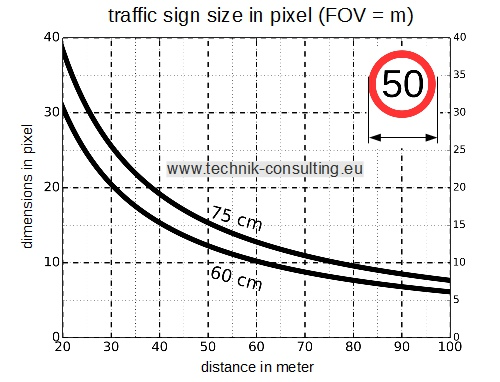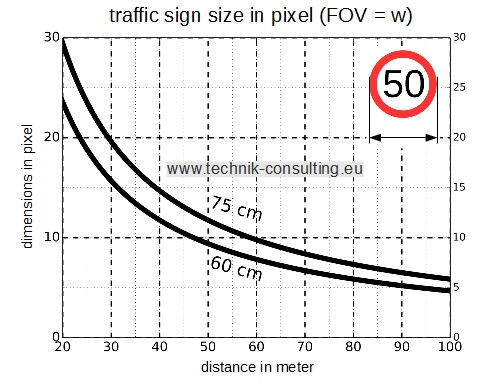Example recording road sign with GoPro
Distance 79 Meter to a road sign with a diameter of 60 cm.
GoPro 3 setting: 1920 pixel x 1080 pixel; FOV: n (narrow)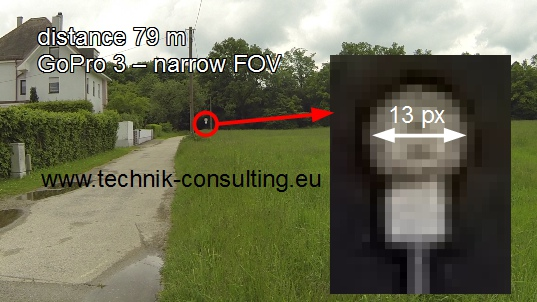From the picture, a pixel width of about 13 pixels results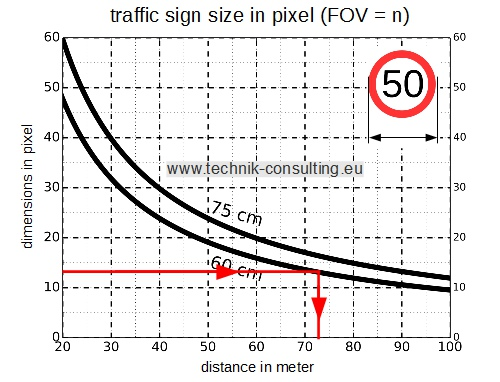From the diagram for the road sign with 60 cm diameter and a FOV of n results in a distance of 73 meters. The measuring inaccuracy in the road sign and the distance can be calculated to be 7 meters. The determined 73 meters are within tolerance. The calculation of the accuracy of measurement is carried out below.

## Accuracy of the range finder or altitude measurement

Do the measuring object not match at the distance or height, the measurement accuracy is limited. If the measurement object in the picture is only a few pixels in size the measurement uncertainty is large.

The smallest measuring unit is a pixel. This is expressed by B. The greater the extent of the object to be measured in pixels, the more precise the measurement.

How precisely you can measure is computable.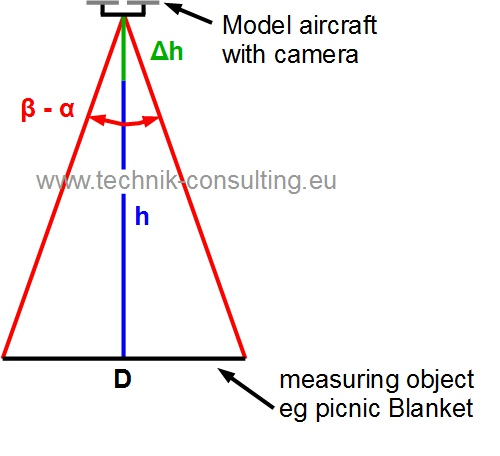If the distance changes by ?h, the angle beta reduced by Alpha. This is at a height gain and corresponds to the practical experience that a more distant object appears smaller. Descent the drone, the angle beta is increased by Alpha. ?h Is negative in this case.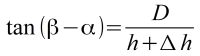At best the object can be measured precisely on a pixel. This is the maximum resolution in the measurement. Interpolations between pixels are not considered.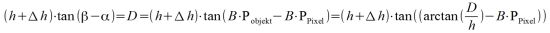This equation can not resolve analytically to D. The numerical solution always works and will be shown later. In order nevertheless to arrive at a useful formula, approximation methods can be used.

## Identify simple formula for calculating the tolerance

For small angles, the angles can be represented as an approximation functions of the Taylor series.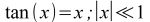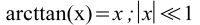This allows the above formula simplify as follows: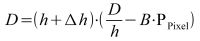After forming and converting the angle in radians (for the approximation formula):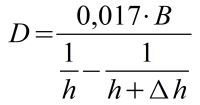D: minimum dimension of the measured object
h: height or distance of the camera to the object
?h: Desired resolution
B: visual angle of one pixel

example GoPro 3 (FOV: m)
With a pixel resolution, one meter accuracy and an altitude of 100 meters there is a minimum object size on the ground of 9.6 meters.

The measuring object should be circular. Deviations from the circular shape are easy to recognize. These could happen at:
• Picture errors (usually by vibration, fisheye lens in the border area).
• No precise positioning over the object, especially with a fisheye lens.

The approximate formula is perfectly adequate for practical purposes.
If a drone is in 100 meters above ground level and with the GoPro camera (FOV: m) follows want measurable to within one meter: The measuring object must have at least a size of 9.6 meters. In border areas may be easier for an average round objects are found, if the diameter is measured from different angles.

## Height measurements with a fisheye lens

If the drone is not exactly over the round measurement object, you should always use the long side of the measuring object. This applies in particular to fisheye lenses.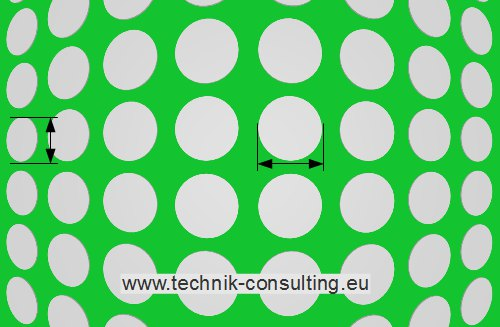example: Round white tablecloths in a meadow.
Better use the longer side, if not the object being measured is in the middle.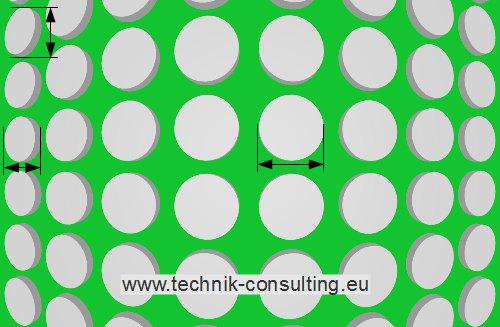Thick or height measurement objects can cause faulty measurements. Measurements left in the picture do not measure the diameter, but also contain partially the height of the measurement object. This gives rise to measurement error.

With the following diagrams, you can easily determine the minimum object size depending on the distance and setting of the GoPro HERO 3camera. The image resolution of the camera needs to set at 1920 pixels x 1020 pixels.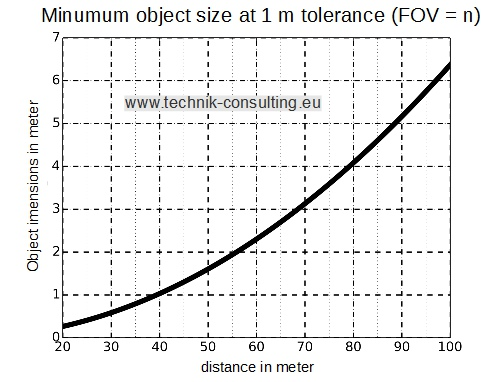If you want to measure exactly 1 meter at 80 meters and a FOV (GoPro 3) of m, the measuring object should be at least 4 meter in size.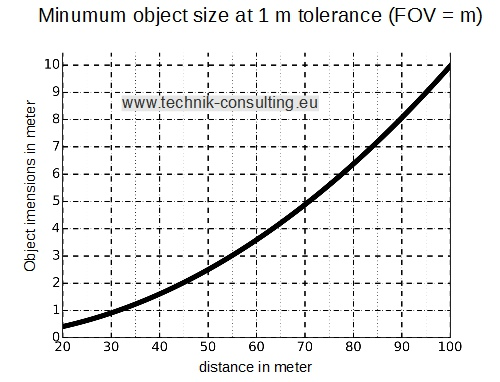If you want to measure exactly 1 meter at 80 meters and a FOV (GoPro 3) of m, the measuring object should be at least 6 meter in size.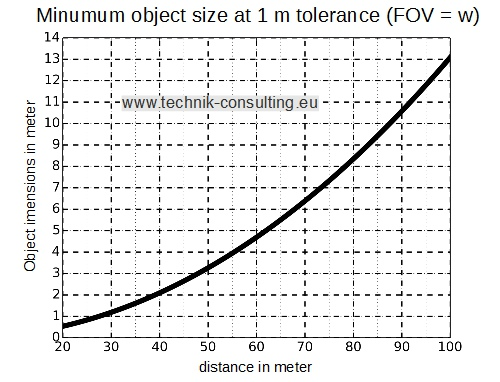If you want to measure exactly 1 meter at 80 meters and a FOV (GoPro 3) of m, the measuring object should be at least 8 meter in size.

## Camera settings for optimal altimeter

Depending on the digital camera it is possible to change the perspective. On the one hand via optical zoom, on the other hand on the sensor itself. The latter is for example used in the GoPro action camera. Depending on the camera settings at the GoPro thus changes the angle of view (FOV - Field of view).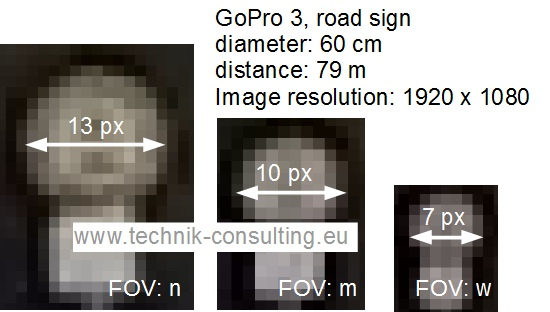Sample images at the same distance and different settings.

The distance measurement by image analysis has high accuracy and measurement rate. With a GoPro action camera can be recorded per second easily 50 good pictures. This corresponds to a measurement time of 20 ms. The high accuracy at high measuring frequency makes it possible to determine more interesting parameters.

## Measure the rate of ascent

If the ascent rate is to be determined by a drone or model helicopter this can be with the image analysis also determined. Let hover the drone obove the measurement object (eg a round picnic blanket) and then climb vertically upwards. Measure the height at a certain times and you can determine the rate of climb. Thus the image analysis is exact, it is recommended that before further processing of the video display image numbers or time. Thus, each still image can be clearly identified later.

## Measuring the vertical acceleration

With the high measurement rate of the image recording the acceleration can be also calculated in addition to the speed. For this purpose, the rise velocity of the drone is calculated at different times. About the change of velocity over time it is easy to calculate the acceleration.

## Comparison of different height measurements

Following are compared with different readings.

The following sensors were used:
1. Altimeter by image analysis (Object size 10 m)
2. Altimeter by image analysis (Object size 1,36 m)
4. eLogger GPS-height
6. eLogger barometer

data set A - measuring
(Height above ground)
1 2 3
calculated height (object 10 m)27 m41 m82 m
calculated height (object 1,36 m)29 m43 m77 m
AQ-GPS-height28 m36 m73 m
eLogger GPS-height26 m34 m58 m
AQ Baro height27 m35 m73 m
eLo height baro32 m53 m94 m

data set B - measuring
(Height above ground)
1 2 3 4
calculated height (object 10 m)4 m15 m40 m100 m
calculated height (object 1,36 m)8 m17 m42 m102 m
AQ-GPS-height7 m38 m64 m127 m
eLogger GPS-height7 m8 m16 m27 m
AQ Baro height6 m37 m63 m126 m
eLo height baro10 m33 m60 m118 m

The sensors, there is a large tolerance, although the measurements were performed at the same time in the drone.

## Conclusion drone altimeter by image analysis with a drone

You can see that with a simple video much data can be obtained via the model aircraft. Whether drone, helicopter or airplane. The measurement is accurate and easy to understand and quickly if properly handled. The measurement can be performed as soon as a line of sight is to the measurement object. Regardless of poor GPS reception or problems with the barometric measurement. For accurate barometric altitude measurement by the way not only the weather has to play along, and the sensor must be installed so that wind or wind generally has no effect. The major disadvantage in the image evaluation is that these can be carried out only after the flight. If no sensors are attached to the flight model, the analysis of the image data is an easy way to determine the altitude of the drone, helicopter or airplane model. Distance measurements for other purposes are also possible. With the image analysis, you can also check whether existing sensors are working properly. Especially under unfavorable conditions provide these values unusable. Think of GPS height measurements near tall buildings or in mountain valleys. The image recording can be carried out immediately after turning on the camera. Wait for valid GPS signals is omitted.

Each measurement method has advantages and disadvantages. What predominate, always depends on the anticipated usage scenario.

The advantages of the height measurement by image analysis are
• No dependency on GPS signals. Particularly in poor reception conditions.
• No dependence on air pressure fluctuations due to weather changes or the wind.
• With a well chosen measuring object very precisely.
• error detection (eg in bad picture) possible.
• High measurement rates of over 50 Hz possible.

• A camera must be mostly carried (extra weight). Alternative, the drone is taken from the ground.
• Only accurate results with fisheye lenses when measured vertically from the measurement object.
• The height data are not immediately available.
• For zoom lenses, a fixed zoom level should remain set.

Good measuring objects
• The measuring object should always be flat. At high measurement objects measuring errors can occur when viewed obliquely.
• A ring is better than a circle. The center of a wide strip can be more easily determined than the edge of a circle.
• Contrast to the environment.
• mat surface. A brilliant film can reflect the environment and cause measurement errors.
• Symmetric, best circular. Error e.g. Distortions can be so recognized.
• big enough. The larger the object being measured, the better the height resolution. There may be a plurality of measurement objects (eg rings) are each other at different heights with high resolution scan. A large ring is not detected, for example, at a low altitude.